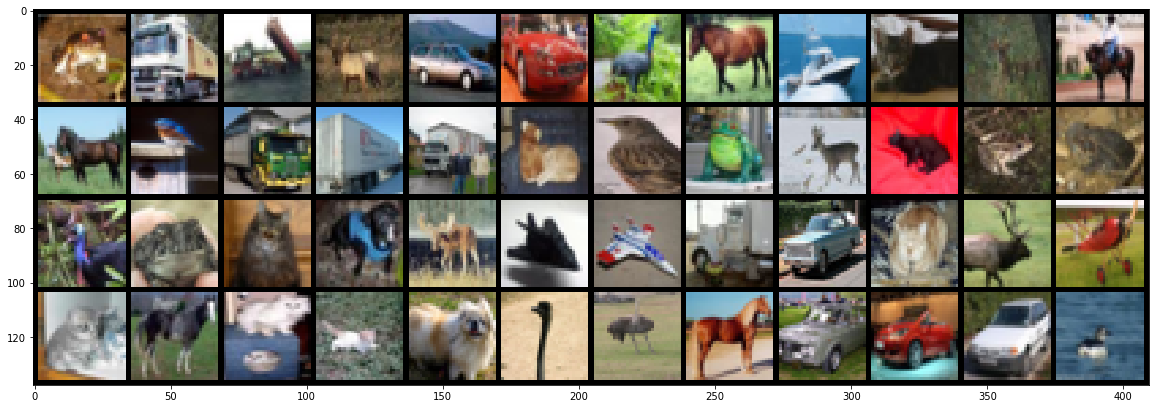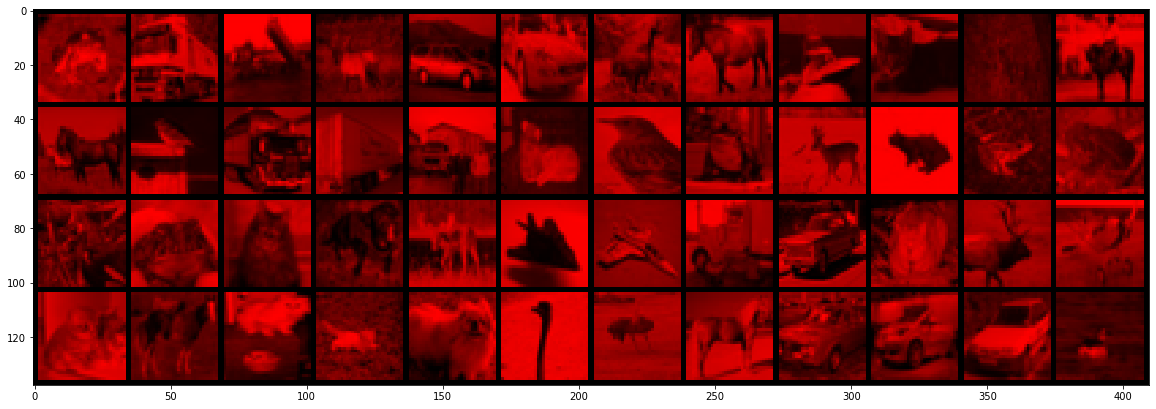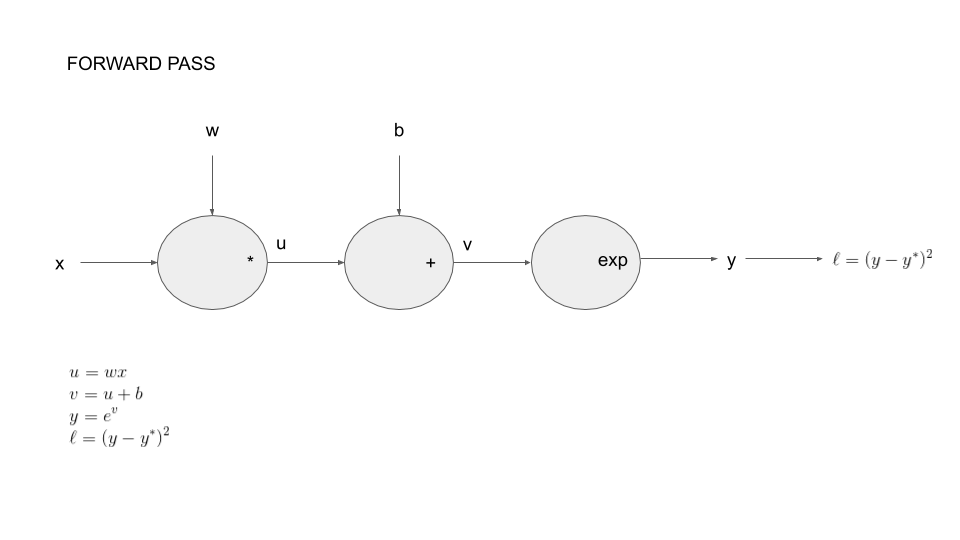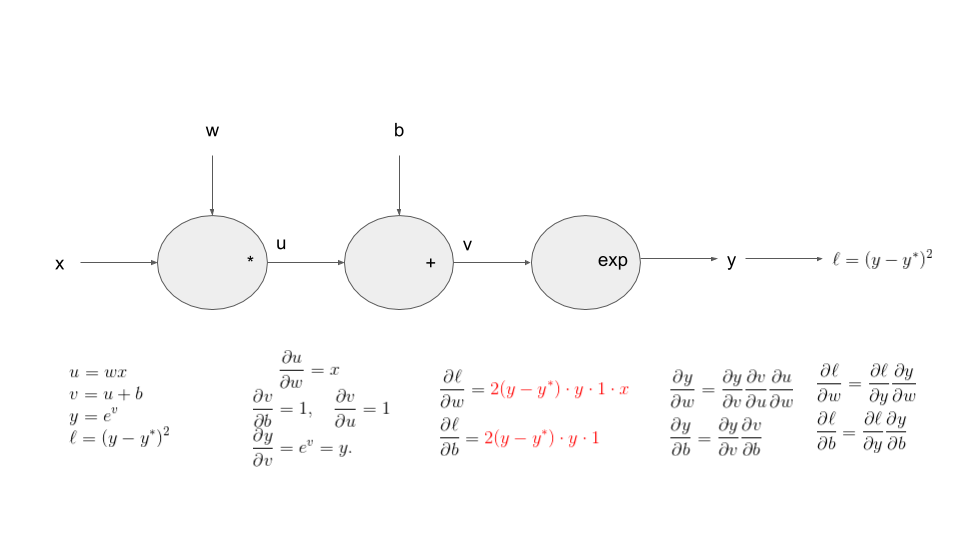This website contains quizzes for the Deep Learning course. Modules on the left correspond to modules on this website.

1 - Take a quiz as soon as you get to it.

2- Do not skip a quiz.

Every quiz looks like the one below. Try it out by clicking "Start".

If you get a question incorrect, you will see the correct answer like above.

Now you are ready to start!# Python

Quiz to test your knowledge of python.

# 3. Lazy evaluation

Now is time to look at some explanations# Module 2: PyTorch tensors and automatic differentiation

You can either do the quizzes corresponding to Module 2 with a static version of the notebook:

or just do all the quizzes

If you did not make any mistake, you can safely go to the end of Module 2b# Module 2: PyTorch tensors and automatic differentiation

Video timestamp

import matplotlib.pyplot as plt
%matplotlib inline
import torch
import numpy as np

> torch.__version__

'1.12.1+cu113'


Tensors are used to encode the signal to process, but also the internal states and parameters of models.

Manipulating data through this constrained structure allows to use CPUs and GPUs at peak performance.

Construct a 3x5 matrix, uninitialized:

x = torch.empty(3,5)
print(x.dtype)
print(x)

torch.float32
tensor([[7.9394e-35, 0.0000e+00, 3.3631e-44, 0.0000e+00,        nan],
[0.0000e+00, 1.1578e+27, 1.1362e+30, 7.1547e+22, 4.5828e+30],
[1.2121e+04, 7.1846e+22, 9.2198e-39, 7.0374e+22, 0.0000e+00]])


If you got an error this stackoverflow link might be useful...

x = torch.randn(3,5)
print(x)

tensor([[-0.0515,  0.6647,  0.5428,  2.5307,  0.9185],
[-0.2556,  0.5543,  1.6044,  0.8425, -1.0667],
[-0.5247,  0.2197,  0.8738,  0.8047,  0.7197]])

print(x.size())

torch.Size([3, 5])


torch.Size is in fact a tuple, so it supports the same operations.

Video timestamp

x.size()

5

x.size() == (3,5)

True


### Bridge to numpy

Video timestamp

y = x.numpy()
print(y)

[[-0.05147836  0.6646777   0.54277486  2.5307057   0.9185137 ]
[-0.25555766  0.55434686  1.6044122   0.8425406  -1.0667061 ]
[-0.52467006  0.21967238  0.87380797  0.8046722   0.7197009 ]]

a = np.ones(5)
b = torch.from_numpy(a)
print(a.dtype)
print(b)

float64
tensor([1., 1., 1., 1., 1.], dtype=torch.float64)

c = b.long()
print(c.dtype, c)
print(b.dtype, b)

torch.int64 tensor([1, 1, 1, 1, 1])
torch.float64 tensor([1., 1., 1., 1., 1.], dtype=torch.float64)

xr = torch.randn(3, 5)
print(xr.dtype, xr)

torch.float32 tensor([[ 0.4959, -0.8126, -0.7801,  0.9866,  0.5365],
[-0.9082,  0.1658, -1.2888, -2.4009,  0.5765],
[-0.4390, -0.6147, -1.3412, -0.0609, -1.0023]])

resb = xr + b
resb

tensor([[ 1.4959,  0.1874,  0.2199,  1.9866,  1.5365],
[ 0.0918,  1.1658, -0.2888, -1.4009,  1.5765],
[ 0.5610,  0.3853, -0.3412,  0.9391, -0.0023]], dtype=torch.float64)

resc = xr + c
resc

tensor([[ 1.4959,  0.1874,  0.2199,  1.9866,  1.5365],
[ 0.0918,  1.1658, -0.2888, -1.4009,  1.5765],
[ 0.5610,  0.3853, -0.3412,  0.9391, -0.0023]])


Be careful with types!

resb == resc

tensor([[False,  True,  True, False,  True],
[ True, False,  True,  True, False],
[False,  True,  True, False,  True]])

torch.set_printoptions(precision=10)

resb[0,1]

tensor(0.1874370575, dtype=torch.float64)

resc[0,1]

tensor(0.1874370575)

resc[0,1].dtype

torch.float32

xr[0,1]

tensor(-0.8125629425)

torch.set_printoptions(precision=4)


Video timestamp

Broadcasting automagically expands dimensions by replicating coefficients, when it is necessary to perform operations.

1. If one of the tensors has fewer dimensions than the other, it is reshaped by adding as many dimensions of size 1 as necessary in the front; then
2. for every mismatch, if one of the two tensor is of size one, it is expanded along this axis by replicating coefficients.

If there is a tensor size mismatch for one of the dimension and neither of them is one, the operation fails.

A = torch.tensor([[1.], [2.], [3.], [4.]])
print(A.size())
B = torch.tensor([[5., -5., 5., -5., 5.]])
print(B.size())
C = A + B

torch.Size([4, 1])
torch.Size([1, 5])

C

tensor([[ 6., -4.,  6., -4.,  6.],
[ 7., -3.,  7., -3.,  7.],
[ 8., -2.,  8., -2.,  8.],
[ 9., -1.,  9., -1.,  9.]])


### In-place modification

Video timestamp

x

tensor([[-0.0515,  0.6647,  0.5428,  2.5307,  0.9185],
[-0.2556,  0.5543,  1.6044,  0.8425, -1.0667],
[-0.5247,  0.2197,  0.8738,  0.8047,  0.7197]])

xr

tensor([[ 0.4959, -0.8126, -0.7801,  0.9866,  0.5365],
[-0.9082,  0.1658, -1.2888, -2.4009,  0.5765],
[-0.4390, -0.6147, -1.3412, -0.0609, -1.0023]])

print(x+xr)

tensor([[ 0.4444, -0.1479, -0.2373,  3.5173,  1.4550],
[-1.1637,  0.7201,  0.3156, -1.5584, -0.4903],
[-0.9636, -0.3950, -0.4674,  0.7438, -0.2826]])

x.add_(xr)
print(x)

tensor([[ 0.4444, -0.1479, -0.2373,  3.5173,  1.4550],
[-1.1637,  0.7201,  0.3156, -1.5584, -0.4903],
[-0.9636, -0.3950, -0.4674,  0.7438, -0.2826]])


Any operation that mutates a tensor in-place is post-fixed with an _

For example: x.fill_(y), x.t_(), will change x.

print(x.t())

tensor([[ 0.4444, -1.1637, -0.9636],
[-0.1479,  0.7201, -0.3950],
[-0.2373,  0.3156, -0.4674],
[ 3.5173, -1.5584,  0.7438],
[ 1.4550, -0.4903, -0.2826]])

x.t_()
print(x)

tensor([[ 0.4444, -1.1637, -0.9636],
[-0.1479,  0.7201, -0.3950],
[-0.2373,  0.3156, -0.4674],
[ 3.5173, -1.5584,  0.7438],
[ 1.4550, -0.4903, -0.2826]])


### Shared memory

Video timestamp

Also be careful, changing the torch tensor modify the numpy array and vice-versa...

This is explained in the PyTorch documentation here: The returned tensor by torch.from_numpy and ndarray share the same memory. Modifications to the tensor will be reflected in the ndarray and vice versa.

a = np.ones(5)
b = torch.from_numpy(a)
print(b)

tensor([1., 1., 1., 1., 1.], dtype=torch.float64)

a = 0
print(b)

tensor([1., 1., 0., 1., 1.], dtype=torch.float64)

b = 5
print(a)

[1. 1. 0. 5. 1.]


### Cuda

Video timestamp

torch.cuda.is_available()

True

#device = torch.device('cpu')
device = torch.device('cuda') # Uncomment this to run on GPU

x.device

device(type='cpu')

# let us run this cell only if CUDA is available
# We will use torch.device objects to move tensors in and out of GPU
if torch.cuda.is_available():
y = torch.ones_like(x, device=device)  # directly create a tensor on GPU
x = x.to(device)                       # or just use strings .to("cuda")
z = x + y
print(z,z.type())
print(z.to("cpu", torch.double))       # .to can also change dtype together!

tensor([[ 1.4444, -0.1637,  0.0364],
[ 0.8521,  1.7201,  0.6050],
[ 0.7627,  1.3156,  0.5326],
[ 4.5173, -0.5584,  1.7438],
[ 2.4550,  0.5097,  0.7174]], device='cuda:0') torch.cuda.FloatTensor
tensor([[ 1.4444, -0.1637,  0.0364],
[ 0.8521,  1.7201,  0.6050],
[ 0.7627,  1.3156,  0.5326],
[ 4.5173, -0.5584,  1.7438],
[ 2.4550,  0.5097,  0.7174]], dtype=torch.float64)

x = torch.randn(1)
x = x.to(device)

x.device

device(type='cuda', index=0)

# the following line is only useful if CUDA is available
x = x.data
print(x)
print(x.item())
print(x.cpu().numpy())

tensor([-0.8821], device='cuda:0')
-0.8821402192115784
[-0.8821402]


# Simple interfaces to standard image data-bases

Video timestamp

An example, the CIFAR10 dataset.

import torchvision

data_dir = 'content/data'

cifar.data.shape

Downloading https://www.cs.toronto.edu/~kriz/cifar-10-python.tar.gz to content/data/cifar-10-python.tar.gz

0%|          | 0/170498071 [00:00<?, ?it/s]

Extracting content/data/cifar-10-python.tar.gz to content/data

(50000, 32, 32, 3)


Documentation about the permute operation.

x = torch.from_numpy(cifar.data).permute(0,3,1,2).float()
x = x / 255
print(x.type(), x.size(), x.min().item(), x.max().item())

torch.FloatTensor torch.Size([50000, 3, 32, 32]) 0.0 1.0


Documentation about the narrow(input, dim, start, length) operation.

# Narrows to the first images, converts to float
x = torch.narrow(x, 0, 0, 48)

x.shape

torch.Size([48, 3, 32, 32])

# Showing images
def show(img):
npimg = img.numpy()
plt.figure(figsize=(20,10))
plt.imshow(np.transpose(npimg, (1,2,0)), interpolation='nearest')

show(torchvision.utils.make_grid(x, nrow = 12))# Kills the green and blue channels
x.narrow(1, 1, 2).fill_(0)
show(torchvision.utils.make_grid(x, nrow = 12))Video timestamp

When executing tensor operations, PyTorch can automatically construct on-the-fly the graph of operations to compute the gradient of any quantity with respect to any tensor involved.

To be more concrete, we introduce the following example: we consider parameters $w\in \mathbb{R}$ and $b\in \mathbb{R}$ with the corresponding function: \begin{eqnarray*} \ell = \left(\exp(wx+b) - y^* \right)^2 \end{eqnarray*}

Our goal here, will be to compute the following partial derivatives: \begin{eqnarray*} \frac{\partial \ell}{\partial w}\mbox{ and, }\frac{\partial \ell}{\partial b}. \end{eqnarray*}

The reason for doing this will be clear when you will solve the practicals for this lesson!

You can decompose this function as a composition of basic operations. This is call the forward pass on the graph of operations.Let say we start with our model in numpy:

w = np.array([0.5])
b = np.array()
xx = np.array([0.5])#np.arange(0,1.5,.5)


transform these into tensor:

xx_t = torch.from_numpy(xx)
w_t = torch.from_numpy(w)
b_t = torch.from_numpy(b)


Video timestamp

A tensor has a Boolean field requires_grad, set to False by default, which states if PyTorch should build the graph of operations so that gradients with respect to it can be computed.

w_t.requires_grad

False


We want to take derivative with respect to $w$ so we change this value:

w_t.requires_grad_(True)

tensor([0.5000], dtype=torch.float64, requires_grad=True)


We want to do the same thing for $b$ but the following line will produce an error!

b_t.requires_grad_(True)

---------------------------------------------------------------------------

RuntimeError                              Traceback (most recent call last)

<ipython-input-49-68842c726fce> in <module>

RuntimeError: only Tensors of floating point dtype can require gradients


Reading the error message should allow you to correct the mistake!

dtype = torch.float64

b_t = b_t.type(dtype)

b_t.requires_grad_(True)

tensor([2.], dtype=torch.float64, requires_grad=True)


Video timestamp

We now compute the function:

def fun(x,ystar):
y = torch.exp(w_t*x+b_t)
print(y)

ystar_t = torch.randn_like(xx_t)
l_t = fun(xx_t,ystar_t)

tensor([9.4877], dtype=torch.float64, grad_fn=<ExpBackward0>)

l_t

tensor(89.3094, dtype=torch.float64, grad_fn=<SumBackward0>)

l_t.requires_grad

True


After the computation is finished, i.e. forward pass, you can call .backward() and have all the gradients computed automatically.

print(w_t.grad)

None

l_t.backward()

print(w_t.grad)

tensor([89.6626], dtype=torch.float64)
tensor([179.3251], dtype=torch.float64)


Video timestamp

Let's try to understand these numbers...yy_t = torch.exp(w_t*xx_t+b_t)
print(torch.sum(2*(yy_t-ystar_t)*yy_t*xx_t))
print(torch.sum(2*(yy_t-ystar_t)*yy_t))

tensor(89.6626, dtype=torch.float64, grad_fn=<SumBackward0>)


tensor.backward() accumulates the gradients in the grad fields of tensors.

l_t = fun(xx_t,ystar_t)
l_t.backward()

tensor([9.4877], dtype=torch.float64, grad_fn=<ExpBackward0>)

print(w_t.grad)

tensor([179.3251], dtype=torch.float64)
tensor([358.6502], dtype=torch.float64)


By default, backward deletes the computational graph when it is used so that you will get an error below:

l_t.backward()

---------------------------------------------------------------------------

RuntimeError                              Traceback (most recent call last)

<ipython-input-62-e295af15a710> in <module>
----> 1 l_t.backward()

/usr/local/lib/python3.7/dist-packages/torch/_tensor.py in backward(self, gradient, retain_graph, create_graph, inputs)
394                 create_graph=create_graph,
395                 inputs=inputs)
397
398     def register_hook(self, hook):

173     Variable._execution_engine.run_backward(  # Calls into the C++ engine to run the backward pass
174         tensors, grad_tensors_, retain_graph, create_graph, inputs,
--> 175         allow_unreachable=True, accumulate_grad=True)  # Calls into the C++ engine to run the backward pass
176

RuntimeError: Trying to backward through the graph a second time (or directly access saved tensors after they have already been freed). Saved intermediate values of the graph are freed when you call .backward() or autograd.grad(). Specify retain_graph=True if you need to backward through the graph a second time or if you need to access saved tensors after calling backward.

# Manually zero the gradients
l_t = fun(xx_t,ystar_t)
l_t.backward(retain_graph=True)
l_t.backward()

tensor([9.4877], dtype=torch.float64, grad_fn=<ExpBackward0>)
tensor([179.3251], dtype=torch.float64)
tensor([358.6502], dtype=torch.float64)


The gradients must be set to zero manually. Otherwise they will cumulate across several .backward() calls. This accumulating behavior is desirable in particular to compute the gradient of a loss summed over several “mini-batches,” or the gradient of a sum of losses.# quizzes only

All quizzes for Module 2:

If something is unclear, you should probably get a refresher here

If something is unclear, you should probably get a refresher here

If something is unclear, you should probably get a refresher here# Module 3: Loss functions for classification

These are the quizzes corresponding to Module 3

In all the questions below we assume that all the import have been done

import torch
import torch.nn as nn


Try to answer the questions without running the code ;-)

We run the following code:

> m = nn.Sigmoid()
> loss = nn.BCELoss()
> target = torch.empty(3).random_(2)
> print(target)
tensor([0., 1., 1.])
> optimizer = torch.optim.SGD([input], lr = 0.1)
> print(m(input))
> for _ in range(10000):
output = loss(m(input), target)
output.backward()
optimizer.step()
> print(xxxxxx)


and obtain the following result:

tensor([0.0030, 0.9970, 0.9970], grad_fn=<SigmoidBackward>)


We run the following code:

> target = torch.empty(1,2,3).random_(2)
> print(target)
tensor([[[1., 1., 1.],
[0., 0., 1.]]])
> optimizer = torch.optim.SGD([input], lr = 0.1)
> print(m(input).size())
torch.Size([1, 2, 3])


We then run

for _ in range(10000):
output = loss(m(input), target)
output.backward()
optimizer.step()


We run the following code:

> target = torch.randn(3)
> print(target)
tensor([-0.1272, -0.4165,  0.1002])
> optimizer = torch.optim.SGD([input], lr = 0.1)
> print(m(input))


and then

for _ in range(10000):
output = loss(m(input), target)
output.backward()
optimizer.step()
print(m(input))


We run the following code:

> target = 10*torch.randn(3)
> print(target)
tensor([ 12.1225, -11.8731,  19.2255])
> optimizer = torch.optim.SGD([input], lr = 0.1)
> print(m(input))


and then

for _ in range(10000):
output = loss(m(input), target)
output.backward()
optimizer.step()
print(m(input))


We run:

> loss2 = nn.BCEWithLogitsLoss()
> target = torch.empty(3).random_(2)
> print(target)
tensor([0., 1., 0.])


and then code1:

input = torch.randn(3, requires_grad=True)
optimizer = torch.optim.SGD([input], lr = 0.1)
for _ in range(500):
output = loss2(m(input), target)
output.backward()
optimizer.step()
print(m(input))


and then code2:

input = torch.randn(3, requires_grad=True)
optimizer = torch.optim.SGD([input], lr = 0.1)
for _ in range(500):
output = loss2(input, target)
output.backward()
optimizer.step()
print(m(input))


We run:

> loss3 = nn.NLLLoss()
> m3 = nn.LogSoftmax(dim=1)
> target = torch.empty(4).random_(6)
> print(target)
tensor([1., 5., 0., 2.])


then code1:

input = torch.randn(4, requires_grad=True)
optimizer = torch.optim.SGD([input], lr = 0.1)
for _ in range(1000):
output = loss3(m3(input), target)
output.backward()
optimizer.step()
print(torch.exp(m3(input)))


code2:

input = torch.randn((4,7), requires_grad=True)
optimizer = torch.optim.SGD([input], lr = 0.1)
for _ in range(1000):
output = loss3(m3(input), target.long())
output.backward()
optimizer.step()
print(torch.exp(m3(input)))


code3:

input = torch.randn((4,6), requires_grad=True)
optimizer = torch.optim.SGD([input], lr = 0.1)
for _ in range(1000):
output = loss3(m3(input), target)
output.backward()
optimizer.step()
print(torch.exp(m3(input)))


We now take

> target = torch.empty(4,2,3).random_(6)
> print(target)
tensor([[[3., 1., 0.],
[4., 2., 3.]],

[[1., 3., 3.],
[0., 1., 0.]],

[[2., 1., 1.],
[0., 4., 5.]],

[[1., 3., 5.],
[3., 2., 5.]]])


We now run

loss4 = nn.CrossEntropyLoss()
m4 = nn.Softmax(dim=1)
target = torch.empty(4).random_(6)
optimizer = torch.optim.SGD([input], lr = 0.1)


then code1

for _ in range(500):
output = loss4(input, target.long())
output.backward()
optimizer.step()
print(m4(input))


code2

for _ in range(500):
output = loss3(input, target.long())
output.backward()
optimizer.step()
print(m4(input))


code3

for _ in range(500):
output = loss3(m3(input), target.long())